NABARD Quantitative Aptitude Practice: 5

# NABARD Quantitative Aptitude Practice: 5

Test Description

## 30 Questions MCQ Test NABARD Assistant Manager Grade B Mock Test Series | NABARD Quantitative Aptitude Practice: 5

NABARD Quantitative Aptitude Practice: 5 for Banking Exams 2022 is part of NABARD Assistant Manager Grade B Mock Test Series preparation. The NABARD Quantitative Aptitude Practice: 5 questions and answers have been prepared according to the Banking Exams exam syllabus.The NABARD Quantitative Aptitude Practice: 5 MCQs are made for Banking Exams 2022 Exam. Find important definitions, questions, notes, meanings, examples, exercises, MCQs and online tests for NABARD Quantitative Aptitude Practice: 5 below.
Solutions of NABARD Quantitative Aptitude Practice: 5 questions in English are available as part of our NABARD Assistant Manager Grade B Mock Test Series for Banking Exams & NABARD Quantitative Aptitude Practice: 5 solutions in Hindi for NABARD Assistant Manager Grade B Mock Test Series course. Download more important topics, notes, lectures and mock test series for Banking Exams Exam by signing up for free. Attempt NABARD Quantitative Aptitude Practice: 5 | 30 questions in 25 minutes | Mock test for Banking Exams preparation | Free important questions MCQ to study NABARD Assistant Manager Grade B Mock Test Series for Banking Exams Exam | Download free PDF with solutions
 1 Crore+ students have signed up on EduRev. Have you?
NABARD Quantitative Aptitude Practice: 5 - Question 1

### Study the pie chart carefully to answer the questions that follow: Percentage breakup of number of children in five different villages and breakup of children attending school from those villages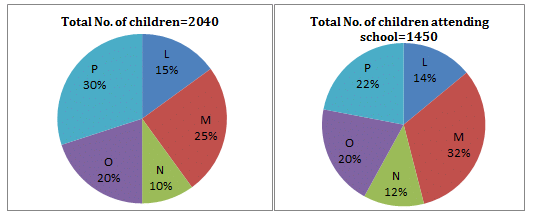Q. What is the respective ratio of total number of children from village O to the number of children attending school from the same village?

Detailed Solution for NABARD Quantitative Aptitude Practice: 5 - Question 1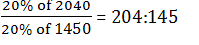NABARD Quantitative Aptitude Practice: 5 - Question 2

### Study the pie chart carefully to answer the questions that follow: Percentage breakup of number of children in five different villages and breakup of children attending school from those villagesQ. What is the number of children attending school from village N?

NABARD Quantitative Aptitude Practice: 5 - Question 3

### Study the pie chart carefully to answer the questions that follow: Percentage breakup of number of children in five different villages and breakup of children attending school from those villagesQ. What is the total number of children not attending school from villages M and N together?

Detailed Solution for NABARD Quantitative Aptitude Practice: 5 - Question 3

No of children in village M and N together
= (25 + 10)% of 2040 = 714
No of children attending school from village M and N together
= (32 + 12)% of 1450 = 638.
Required No. of Children = 714 –638 = 76

NABARD Quantitative Aptitude Practice: 5 - Question 4

Study the pie chart carefully to answer the questions that follow:

Percentage breakup of number of children in five different villages and breakup of children attending school from those villagesQ. What is the total number of children from villages P and M together?

NABARD Quantitative Aptitude Practice: 5 - Question 5

Study the pie chart carefully to answer the questions that follow:

Percentage breakup of number of children in five different villages and breakup of children attending school from those villagesQ. The number of children attending school from village L is approximately what percentage of the number of children from that village?

Detailed Solution for NABARD Quantitative Aptitude Practice: 5 - Question 5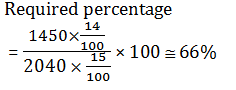NABARD Quantitative Aptitude Practice: 5 - Question 6

Study the table carefully to answer the questions that follow:

Number of people (in thousands) staying in 6 different cities and the percentage of men,women and children in those cities.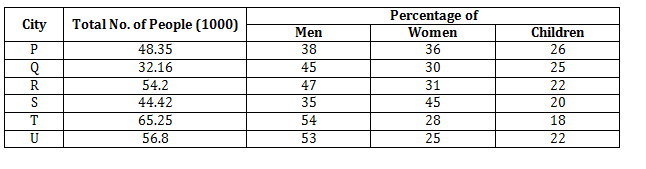Q. What is the ratio of the number of women from city R to that from city T?

Detailed Solution for NABARD Quantitative Aptitude Practice: 5 - Question 6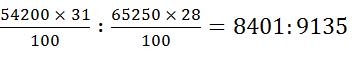NABARD Quantitative Aptitude Practice: 5 - Question 7

Study the table carefully to answer the questions that follow:

Number of people (in thousands) staying in 6 different cities and the percentage of men,women and children in those cities.Q. Which city has the lowest number of children?

Detailed Solution for NABARD Quantitative Aptitude Practice: 5 - Question 7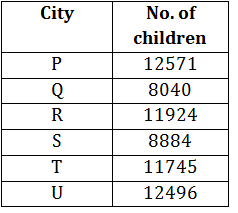NABARD Quantitative Aptitude Practice: 5 - Question 8

Study the table carefully to answer the questions that follow:

Number of people (in thousands) staying in 6 different cities and the percentage of men,women and children in those cities.Q. Total number of people from city U form approximately what per cent of the total number of people from all cities together?

Detailed Solution for NABARD Quantitative Aptitude Practice: 5 - Question 8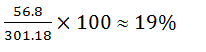NABARD Quantitative Aptitude Practice: 5 - Question 9

Study the table carefully to answer the questions that follow:

Number of people (in thousands) staying in 6 different cities and the percentage of men,women and children in those cities.Q. The number of women from city S forms what per cent of that from city P? (Rounded off to two digits after decimal)

Detailed Solution for NABARD Quantitative Aptitude Practice: 5 - Question 9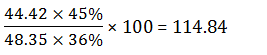NABARD Quantitative Aptitude Practice: 5 - Question 10

Study the table carefully to answer the questions that follow:
Number of people (in thousands) staying in 6 different cities and the percentage of men,women and children in those cities.Q. What is the average number of men from all the cities together?

Detailed Solution for NABARD Quantitative Aptitude Practice: 5 - Question 10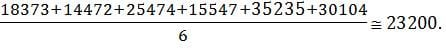NABARD Quantitative Aptitude Practice: 5 - Question 11

What will come in place of the question mark (?) in the following number series ?

3, 10, 32, 100, ?

Detailed Solution for NABARD Quantitative Aptitude Practice: 5 - Question 11

The pattern is : ×3 + 1, ×3 + 2, × 3 + 4, × 3 + 8

NABARD Quantitative Aptitude Practice: 5 - Question 12

What will come in place of the question mark (?) in the following number series ?

5, 3, 4, ?, 38

Detailed Solution for NABARD Quantitative Aptitude Practice: 5 - Question 12

The pattern is : × 1 – 2, × 2 – 2, × 3 –2, × 4 – 2

NABARD Quantitative Aptitude Practice: 5 - Question 13

What will come in place of the question mark (?) in the following number series ?

5, 6, ?, 57, 244

Detailed Solution for NABARD Quantitative Aptitude Practice: 5 - Question 13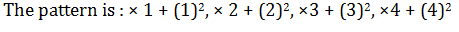NABARD Quantitative Aptitude Practice: 5 - Question 14

What will come in place of the question mark (?) in the following number series ?

3, 10, 21, ?, 51

Detailed Solution for NABARD Quantitative Aptitude Practice: 5 - Question 14

+7, +11, +13, +17.

NABARD Quantitative Aptitude Practice: 5 - Question 15

What will come in place of the question mark (?) in the following number series ?

5, 11, ?, 55, 117

Detailed Solution for NABARD Quantitative Aptitude Practice: 5 - Question 15

The pattern is : × 2 + 1, × 2 + 3, × 2 + 5, × 2 + 7

NABARD Quantitative Aptitude Practice: 5 - Question 16

Two equations (I) and (II) are given in each question. On the basis of these equations you have to decide the relation between ‘x’ and ‘y’ and give answer.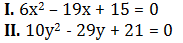Detailed Solution for NABARD Quantitative Aptitude Practice: 5 - Question 16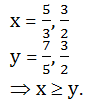NABARD Quantitative Aptitude Practice: 5 - Question 17

Two equations (I) and (II) are given in each question. On the basis of these equations you have to decide the relation between ‘x’ and ‘y’ and give answer.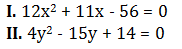Detailed Solution for NABARD Quantitative Aptitude Practice: 5 - Question 17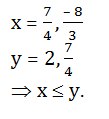NABARD Quantitative Aptitude Practice: 5 - Question 18

Two equations (I) and (II) are given in each question. On the basis of these equations you have to decide the relation between ‘x’ and ‘y’ and give answer.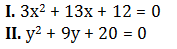Detailed Solution for NABARD Quantitative Aptitude Practice: 5 - Question 18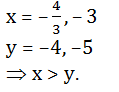NABARD Quantitative Aptitude Practice: 5 - Question 19

Two equations (I) and (II) are given in each question. On the basis of these equations you have to decide the relation between ‘x’ and ‘y’ and give answer.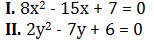Detailed Solution for NABARD Quantitative Aptitude Practice: 5 - Question 19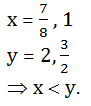NABARD Quantitative Aptitude Practice: 5 - Question 20

Two equations (I) and (II) are given in each question. On the basis of these equations you have to decide the relation between ‘x’ and ‘y’ and give answer.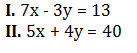Detailed Solution for NABARD Quantitative Aptitude Practice: 5 - Question 20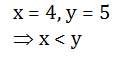NABARD Quantitative Aptitude Practice: 5 - Question 21

A house and a shop were sold for Rs. 1 lakh each, In this transaction, the house sale resulted into 20% loss whereas the shop sale into 20% profit. The entire transaction resulted in :

Detailed Solution for NABARD Quantitative Aptitude Practice: 5 - Question 21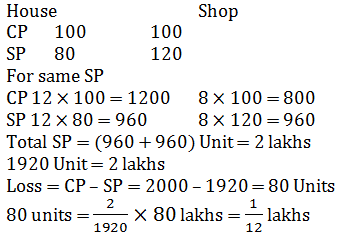NABARD Quantitative Aptitude Practice: 5 - Question 22

The marked price of an article is 50% above cost price. When marked price is increased by 20% and selling price is increased by 20%, the profit doubles. If original marked price is Rs. 300, then original selling price is

Detailed Solution for NABARD Quantitative Aptitude Practice: 5 - Question 22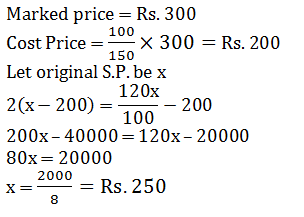NABARD Quantitative Aptitude Practice: 5 - Question 23

A boy aged 12 years is left with Rs. 100,000 which is under a trust. The trustees invest the money at 6% per annum and pay the minor boy a sum of Rs. 2500, for his pocket money at the end of each year. The expenses of trust come out to be Rs. 500 per annum. Find the amount that will be handed over to the minor boy after he attains the age of 18 years.

Detailed Solution for NABARD Quantitative Aptitude Practice: 5 - Question 23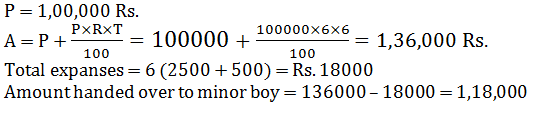NABARD Quantitative Aptitude Practice: 5 - Question 24

Sita deposited Rs. 5,000 at 10% simple interest for 2 years. How much more money will Sita have in her account at the end of two years. If it is compounded semiannually.

Detailed Solution for NABARD Quantitative Aptitude Practice: 5 - Question 24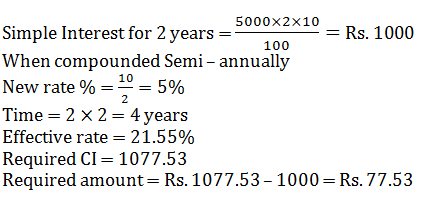NABARD Quantitative Aptitude Practice: 5 - Question 25

Three vessels whose capacities are in the ratio of 3 : 2 : 1 are completely filled with milk and water. The ratio of milk and water in the mixture of vessels are 5 : 2, 4 : 1 and 4 : 1 respectively. Taking 1/3 of first, ½ of second and 1/7 of third mixtures, a new mixture kept in a new vessel is prepared. The percentage of water in the new mixture is

Detailed Solution for NABARD Quantitative Aptitude Practice: 5 - Question 25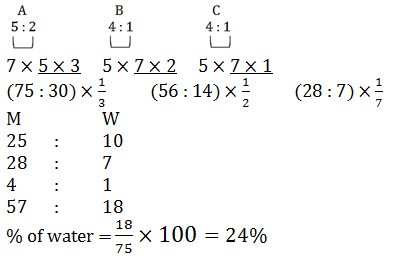NABARD Quantitative Aptitude Practice: 5 - Question 26

In an innings of a cricket match, three players A, B and C scored a total of 361 runs. If the ratio of the number of runs scored by A to that scored by B and also number of runs scored by B to that scored by C be 3 : 2, the number of runs scored by A was

Detailed Solution for NABARD Quantitative Aptitude Practice: 5 - Question 26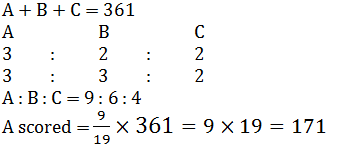NABARD Quantitative Aptitude Practice: 5 - Question 27

A container contains two liquids A and B in the ratio 7 : 5. When 9 litres of mixture are drawn off and the container is filled with B, the ratio of A and B becomes 1 : 1. How many litres of liquid A was in the container initially?

Detailed Solution for NABARD Quantitative Aptitude Practice: 5 - Question 27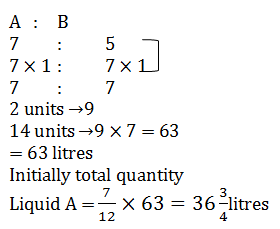NABARD Quantitative Aptitude Practice: 5 - Question 28

P can complete 1/4 of a work in 10 days, Q can complete 40% of the same work in 145 days. R, completes 1/3 of the work in 13 days and S can complete 1/6 of the work in 7 days. Who will be able to complete the work first?

NABARD Quantitative Aptitude Practice: 5 - Question 29

A contractor undertakes to make a road in 40 days and employes 25 men. After 24 days, he finds that only one-third of the road is made. How many extra men should he employ so that he is able to complete the work 4 days earlier?

Detailed Solution for NABARD Quantitative Aptitude Practice: 5 - Question 29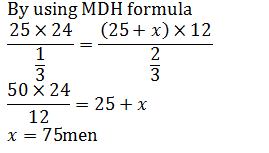NABARD Quantitative Aptitude Practice: 5 - Question 30

A water reservoir has two inlets and one outlet. Through the inlet it can be filled in 3 hours and 3 hours 45 minutes respectively. It can be emptied completely in 1 hour by the outlet. If the two inlets are opened at 01 : 00 pm and 02 : 00 pm respectively and the outlet at 03 : 00 pm then it will be emptied at.

Detailed Solution for NABARD Quantitative Aptitude Practice: 5 - Question 30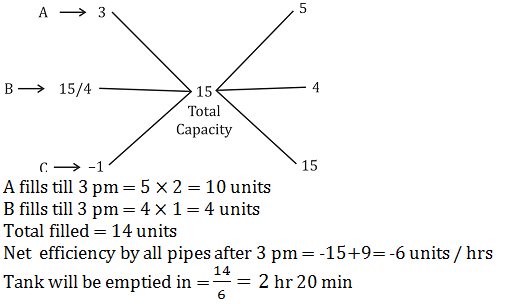## NABARD Assistant Manager Grade B Mock Test Series

1 docs|25 tests
 Use Code STAYHOME200 and get INR 200 additional OFF Use Coupon Code
Information about NABARD Quantitative Aptitude Practice: 5 Page
In this test you can find the Exam questions for NABARD Quantitative Aptitude Practice: 5 solved & explained in the simplest way possible. Besides giving Questions and answers for NABARD Quantitative Aptitude Practice: 5, EduRev gives you an ample number of Online tests for practice

1 docs|25 tests